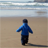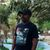# New to Qlik Sense

If you’re new to Qlik Sense, start with this Discussion Board and get up-to-speed quickly.

Announcements
cancel
Showing results for
Did you mean:Creator

## YTD Calculation

Hi All,

I am new to qlik sense.

I have a requirement to calculate YTD.

I have two Fields

The Link_Fiscal_Period has values from 2018001/2018002/2018003 and so on....

The LINK_FISCAL_PERIOD_DATE has values from 'Apr-2017'/May-2017/Jun-2017 and so on..

I wrote an expression to calculate YTD as below but expression is not resulting any values.

Could you please check and let me know is there any issue with expression or do the expression requires any tweaks to get the desired values.

Note : LINK_FISCAL_PERIOD date format is CCYY0MM

The Mapping of these fields are as below:

if(FISCAL_YEAR_PERIOD = '2018001', 'Apr-2017',

if(FISCAL_YEAR_PERIOD = '2018002', 'May-2017',

if(FISCAL_YEAR_PERIOD = '2018003', 'Jun-2017',

if(FISCAL_YEAR_PERIOD = '2018004', 'Jul-2017',

if(FISCAL_YEAR_PERIOD = '2018005', 'Aug-2017',

if(FISCAL_YEAR_PERIOD = '2018006', 'Sep-2017',

if(FISCAL_YEAR_PERIOD = '2018007', 'Oct-2017',

if(FISCAL_YEAR_PERIOD = '2018008', 'Nov-2017',

if(FISCAL_YEAR_PERIOD = '2018009', 'Dec-2017',

if(FISCAL_YEAR_PERIOD = '2018010', 'Jan-2018',

if(FISCAL_YEAR_PERIOD = '2018011', 'Feb-2018',

if(FISCAL_YEAR_PERIOD = '2018012', 'Mar-2018',

if(FISCAL_YEAR_PERIOD = '2019001', 'Apr-2018',

if(FISCAL_YEAR_PERIOD = '2019002', 'May-2018',

if(FISCAL_YEAR_PERIOD = '2019003', 'Jun-2018',

if(FISCAL_YEAR_PERIOD = '2019004', 'Jul-2018',

if(FISCAL_YEAR_PERIOD = '2019005', 'Aug-2018',

if(FISCAL_YEAR_PERIOD = '2019006', 'Sep-2018',

if(FISCAL_YEAR_PERIOD = '2019007', 'Oct-2018',

if(FISCAL_YEAR_PERIOD = '2019008', 'Nov-2018',

if(FISCAL_YEAR_PERIOD = '2019009', 'Dec-2018',

if(FISCAL_YEAR_PERIOD = '2019010', 'Jan-2019',

if(FISCAL_YEAR_PERIOD = '2019011', 'Feb-2019',

if(FISCAL_YEAR_PERIOD = '2019012', 'Mar-2019',

Thanks,

S k

10 RepliesCreator
Author

Hi All,

Did anyone had a chance to look into above requirement

Thanks,Creator III

share sample qvfCreator
Author

Hi,

I cant share the app as it is huge and the data is coming from different sources.

Thanks,

S kCreator
Author

Hi All,

I have now a new requirement.

In the below script i have mappend the dates from data source to fiscal month year.

My fiscal year starts from apr to march.

In the below example when the date is 2018001 to 20180012 then i have mapped to Apr-2017 to Mar-2018 creating my fiscal year. I have hard coded the dates to form fiscal year. Is there way to make the script more dynamic rather than hardcode.

if(FISCAL_YEAR_PERIOD = '2018001', 'Apr-2017',

if(FISCAL_YEAR_PERIOD = '2018002', 'May-2017',

if(FISCAL_YEAR_PERIOD = '2018003', 'Jun-2017',

if(FISCAL_YEAR_PERIOD = '2018004', 'Jul-2017',

if(FISCAL_YEAR_PERIOD = '2018005', 'Aug-2017',

if(FISCAL_YEAR_PERIOD = '2018006', 'Sep-2017',

if(FISCAL_YEAR_PERIOD = '2018007', 'Oct-2017',

if(FISCAL_YEAR_PERIOD = '2018008', 'Nov-2017',

if(FISCAL_YEAR_PERIOD = '2018009', 'Dec-2017',

if(FISCAL_YEAR_PERIOD = '2018010', 'Jan-2018',

if(FISCAL_YEAR_PERIOD = '2018011', 'Feb-2018',

if(FISCAL_YEAR_PERIOD = '2018012', 'Mar-2018',

if(FISCAL_YEAR_PERIOD = '2019001', 'Apr-2018',

if(FISCAL_YEAR_PERIOD = '2019002', 'May-2018',

if(FISCAL_YEAR_PERIOD = '2019003', 'Jun-2018',

if(FISCAL_YEAR_PERIOD = '2019004', 'Jul-2018',

if(FISCAL_YEAR_PERIOD = '2019005', 'Aug-2018',

if(FISCAL_YEAR_PERIOD = '2019006', 'Sep-2018',

if(FISCAL_YEAR_PERIOD = '2019007', 'Oct-2018',

if(FISCAL_YEAR_PERIOD = '2019008', 'Nov-2018',

if(FISCAL_YEAR_PERIOD = '2019009', 'Dec-2018',

if(FISCAL_YEAR_PERIOD = '2019010', 'Jan-2019',

if(FISCAL_YEAR_PERIOD = '2019011', 'Feb-2019',

if(FISCAL_YEAR_PERIOD = '2019012', 'Mar-2019

Note : FISCAL_YEAR_PERIOD date format is YYYY0MM

I am not using master calendar in my app.

Thanks,

SkMVP

felipedl‌  Can have any idea?Master

Modify the script from this:

https://community.qlik.com/docs/DOC-7094

And create expression like this:

Sum({\$<[number]={'*SINV*'},[res_partner.name]={*}-{''},[date_invoice]={'>=\$(YearStart(\$(CurrMonth),0,10))<=\$(MonthEnd(\$(CurrMonth)))'}>}amount_untaxed)Creator
Author

Thanks.

Cant we make the script dynamic without master calander.

The date format that I have YYYY0MM.Creator
Author

Hi All,

Any luck??

Thanks,

S kPartner

I would always have a number representation of the date on the table for this situation, since set analysis will be simpler with number rather than the string.

On your table, do the fiscal year and a numeric date and change the set analysis to:

Calendar:

Date,

num(Date) as NumDate

From [Whatever];

And the expression:

=

sum

(

{<

// Ignore the selected date

Date,

[Cost Type] = {'Total Opex'}

>}

AMOUNT_COA_CRCY_SIGNS

)

/

1000Tags